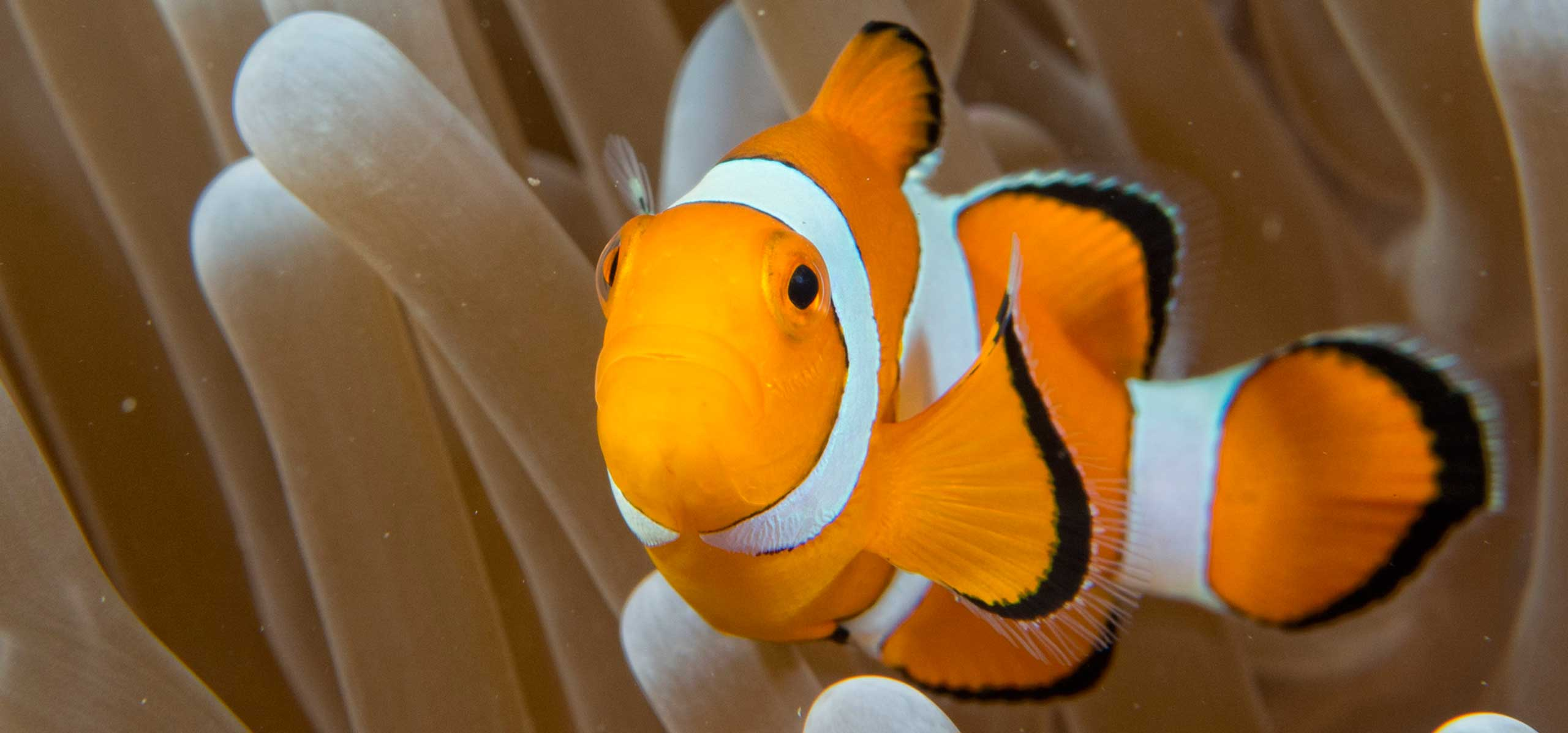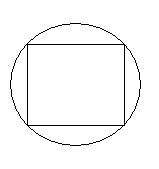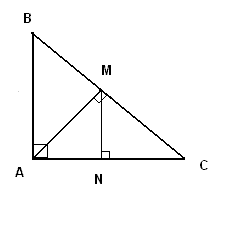Grade 9 maths problems with answers are presented. Solutions and detailed explanations are also included.

1. Which number(s) is(are) equal to its (their) square?
2. Which number(s) is(are) equal to half its (their) square?
3. Which number(s) is(are) equal to the quarter of its (their) square?
4. A car travels from A to B at a speed of 40 mph then returns, using the same road, from B to A at a speed of 60 mph. What is the average speed for the round trip?
5. Tom travels 60 miles per hour going to a neighboring city and 50 miles per hour coming back using the same road. He drove a total of 5 hours away and back. What is the distance from Tom's house to the city he visited?(round your answer to the nearest mile).
6. At 11:00 a.m., John started driving along a highway at constant speed of 50 miles per hour. A quarter of an hour later, Jimmy started driving along the same highway in the same direction as John at the constant speed of 65 miles per hour. At what time will Jimmy catch up with John?
7. Find an equation of the line containing (- 4,5) and perpendicular to the line 5x - 3y = 4.
8. A rectangle field has an area of 300 square meters and a perimeter of 80 meters. What are the length and width of the field?
9. Find the area of a trapezoid whose parallel sides are 12 and 23 centimeters respectively.
10. A rectangular garden in Mrs Dorothy's house has a length of 100 meters and a width of 50 meters. A square swimming pool is to be constructed inside the garden. Find the length of one side of the swimming pool if the remaining area (not occupied by the pool) is equal to one half the area of the rectangular garden.
11. ABC is an equilateral triangle with side length equal to 50 cm. BH is perpendicular to AC. MN is parallel to AC. Find the area of triangle BMN if the length of MN is equal to 12 cm..

12. The height h of water in a cylindrical container with radius r = 5 cm is equal to 10 cm. Peter needs to measure the volume of a stone with a complicated shape and so he puts the stone inside the container with water. The height of the water inside the container rises to 13.2 cm. What is the volume of the stone in cubic cm?.

13. In the figure below the square has all its vertices on the circle. The area of the square is equal to 400 square cm. What is the area of the circular shape?.

14. The numbers 2 , 3 , 5 and x have an average equal to 4. What is x?
15. The numbers x , y , z and w have an average equal to 25. The average of x , y and z is equal to 27. Find w.
16. Find x , y , z so that the numbers 41 , 46 , x , y , z have a mean of 50 and a mode of 45.
17. A is a constant. Find A such that the equation 2x + 1 = 2A + 3(x + A) has a solution at x = 2.
18. 1 liter is equal to 1 cubic decimeter and 1 liter of water weighs 1 kilogram. What is the weight of water contained in a cylindrical container with radius equal to 50 centimeters and height equal to 1 meter?
19. In the figure below triangle ABC is an isosceles right triangle. AM is perpendicular to BC and MN is perpendicular to AC. Find the ratio of the area of triangle MNC to the area of triangle ABC..

20. Pump A can fill a tank of water in 4 hours. Pump B can fill the same tank in 6 hours. Both pumps are started at 8:00 a.m. to fill the same empty tank. An hour later, pump B breaks down and took one hour to repair and was restarted again. When will the tank be full? (round your answer to the nearest minute).
21. Are the lines with equations 2x + y = 2 and x - 2y = 0 parallel, perpendicular or neither?
22. What are the dimensions of the square that has the perimeter and the area equal in value?
23. Find the dimensions of the rectangle that has a length 3 meters more that its width and a perimeter equal in value to its area?
24. Find the circumference of a circular disk whose area is 100π square centimeters.
25. The semicircle of area 50 π centimeters is inscribed inside a rectangle. The diameter of the semicircle coincides with the length of the rectangle. Find the area of the rectangle.
26. A triangle has an area of 200 cm2. Two sides of this triangle measure 26 and 40 cm respectively. Find the exact value of the third side.

Solutions and detailed explanations are also included.

## Answers to the Above Problems

1. 0 , 1
2. 0 , 2
3. 0 , 4
4. 48 miles per hour
5. 136 miles
6. 12:05 p.m.
7. 5y + 3x = 13
8. length = 30 meters , width = 10 meters
9. not enough information to solve the problem.
10. 50 meters
11. 72 √3 square centimeters.
12. 80 π cubic centimeters
13. 200π square centimeters
14. x = 6
15. w = 19
16. x = 45 , y = 45 and z = 73
17. A = - 1/5
18. 250π kilograms
19. 1:4
20. 10:48 a.m.
21. perpendicular
22. square with side length = 4 units
23. length = 6 units and width = 3 units
24. 20π centimeters
25. 200 square centimeters
26. two solutions: 2 √ (89) and 2 √ (1049)